# Double Atwood's Machine

KingBreak
Homework Statement:
A mass M1 is suspended from one end of a rope which is guided over a roller A. The other end carries a second roller of mass M2 that carries a rope with the masses m1 and m2 attached to its ends. The gravitational force is acting on all masses. Calculate the acceleration of the masses m1 and m2 as well as the tension T1 to show that (look at picture)

i've tried to solve by calculating the acceleration on mass m3, but it didn't go well (perhaps my algebra isn't that good lol)... is it possible to solve using a3 ? and if not, how do i solve by calculating a1,a2,t1?

my notation: m1=m1, m2=m2, M1=m3, M2=m4, T1 = T1, T= T2
Relevant Equations:
F=ma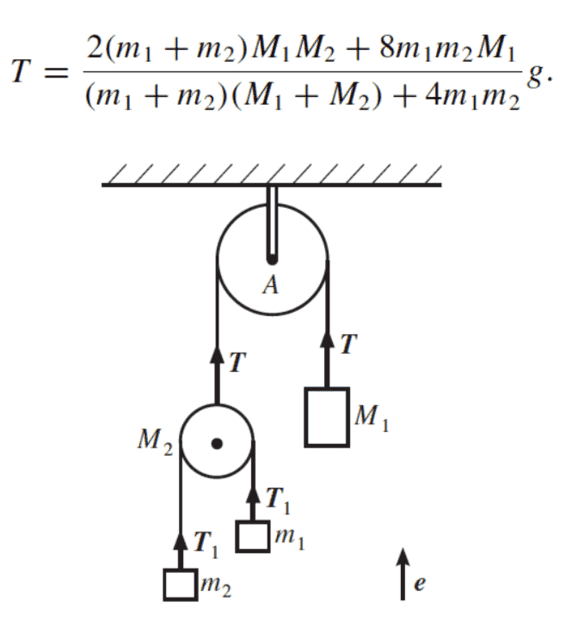My Attempt to solve the problem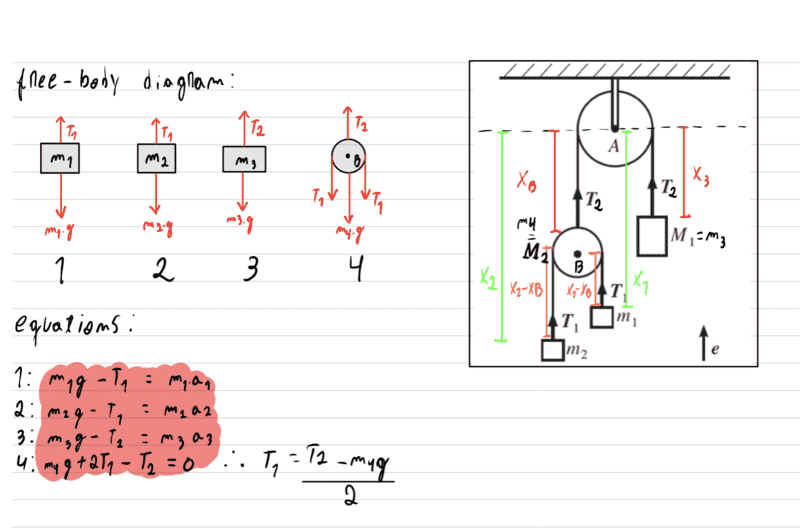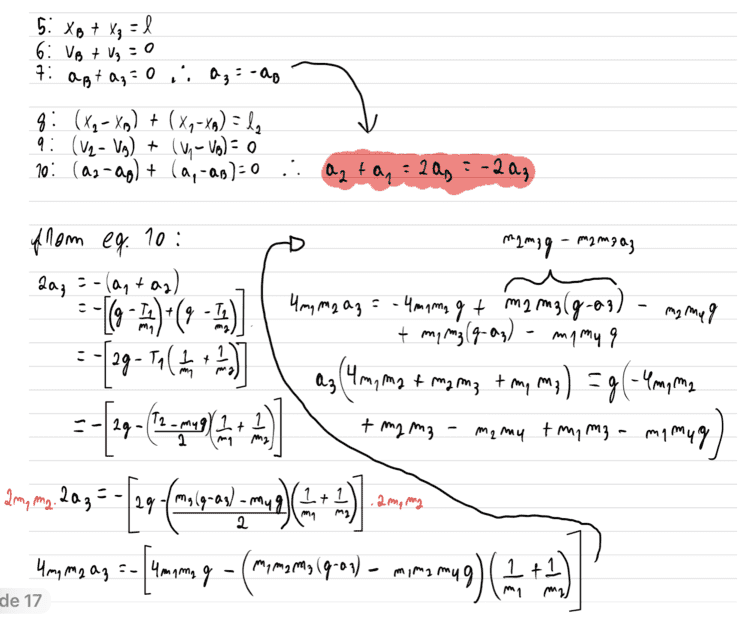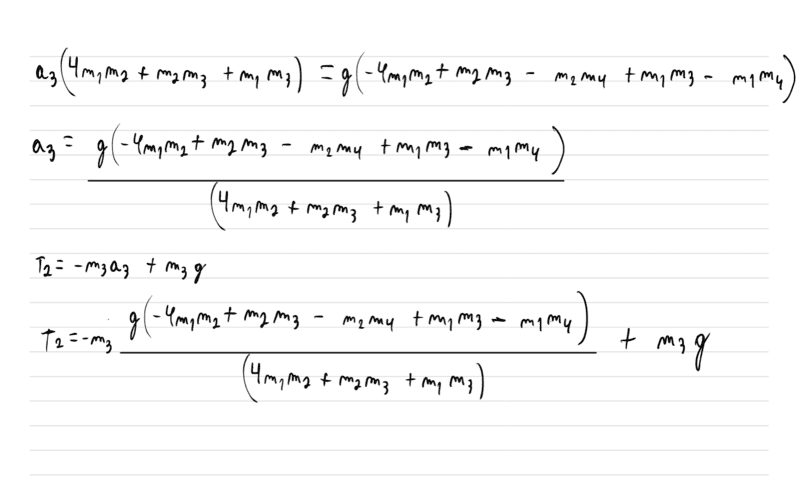Last edited:

Homework Helper
Gold Member
2022 Award
Some of the subscripts are impossible to read in your images. Please post algebra as typed in, per forum rules. Images are for diagrams and textbook extracts.
Your equation (4) seems to assume M2 is not accelerating.

I note that the diagram supplied with the question shows T1 both sides of M2. That looks like a blunder. Assuming M2 has uniformly distributed mass, and the rope does not slip on the pulleys, the rotational inertia of M2 leads to a computably different tension each side. (The radius is irrelevant.)
Likewise A, but in that case we are not given its mass.

•KingBreak
Homework Helper
Gold Member
I note that the diagram supplied with the question shows T1 both sides of M2. That looks like a blunder. Assuming M2 has uniformly distributed mass, and the rope does not slip on the pulleys, the rotational inertia of M2 leads to a computably different tension each side. (The radius is irrelevant.)
Likewise A, but in that case we are not given its mass.
According to my calculations, the final formula, as well as the original figure, work out correctly if you assume that the pulley $M_2$ has all its mass concentrated in its center. In other words, yes, the pulley has mass, but it has zero moment of inertia. There's no need to worry about angular acceleration and such for this problem.

@KingBreak, Welcome to PF!To get you started, you'll first need to form 6 simultaneous equations. From the looks of your work, I think you only started with 4 equations. There are additional constraints that you can use to your advantage. One is a constraint between $m_3$ and $m_4$ (or $M_1$ and $M_2$ using your coursework notation). The other constraint is between $m_1, m_2$ and $m_4$ (or $m_1, m_2,$ and $M_2$ using the coursework notation). [Edit: also, as @haruspex pointed out, you seem to have treated $m_4$ (or $M_2$ using your coursework's notation) as stationary. It is not. That pulley can accelerate linearly too. You'll need to fix that.]

Also, I think that $e$ with the up-arrow ($\uparrow e$) might be trying to tell you that your instructor wants up to be the positive direction. It looks to me in your equations that you chose down as the positive direction. You should probably change that to be consistent with the course-work's intentions. It shouldn't change the final answer, but leaving down as positive might make it more difficult for your instructor to follow your work.

Then, by using you favorite method of eliminating variables, you'll need to eliminate $a_1, a_2, a_3, a_4,$ and $T_1$. You'll need to eliminate these variables carefully such that you leave only $T$, the masses, and $g$ in the final equation.

I won't sugar-coat it for you, there is a lot of algebra in this problem. But it is possible (at least according to my calculations).

Last edited:
•KingBreak and Delta2
Homework Helper
Gold Member
2022 Award
if you assume that the pulley M2 has all its mass concentrated in its center
Sure, but that unexpected circumstance should be stated, not left to be inferred from the variable labels in a diagram. That's why I say it looks like a blunder; the problem setter seems not to have considered it.

•KingBreak
KingBreak
@KingBreak, Welcome to PF!To get you started, you'll first need to form 6 simultaneous equations. From the looks of your work, I think you only started with 4 equations. There are additional constraints that you can use to your advantage. One is a constraint between $m_3$ and $m_4$ (or $M_1$ and $M_2$ using your coursework notation). The other constraint is between $m_1, m_2$ and $m_4$ (or $m_1, m_2,$ and $M_2$ using the coursework notation). [Edit: also, as @haruspex pointed out, you seem to have treated $m_4$ (or $M_2$ using your coursework's notation) as stationary. It is not. That pulley can accelerate linearly too. You'll need to fix that.]
Thank you, Sir!😁

i don't know if I'm doing this right, but i found these other two equations from the relation between accelerations. Is it correct ?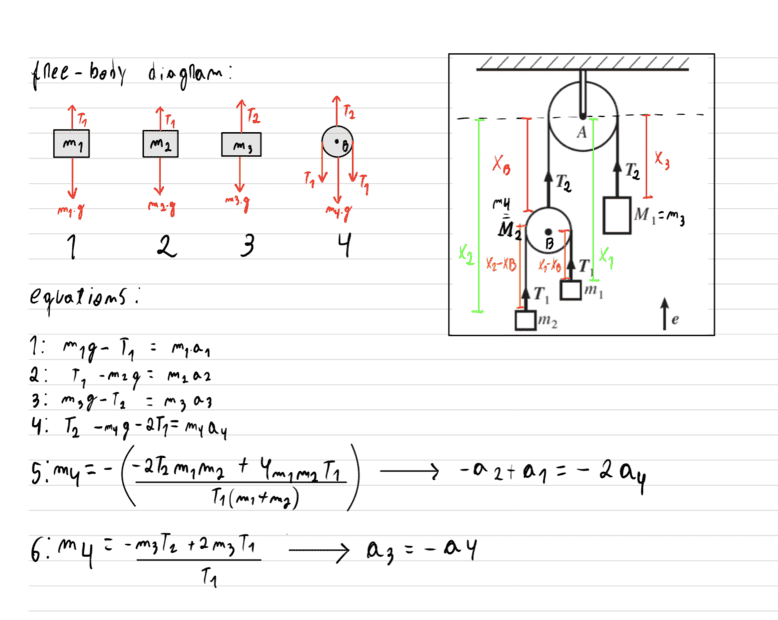Homework Helper
Gold Member
2022 Award
Thank you, Sir!😁

i don't know if I'm doing this right, but i found these other two equations from the relation between accelerations. Is it correct ?View attachment 286115
It's not possible to verify your equations without knowing how your variables are defined. Looks like you are taking some accelerations as positive up and others as positive down.

•collinsmark
KingBreak
Looks like you are taking some accelerations as positive up and others as positive down.
I don't have much information, so I'm assuming that if the object is higher than the one to the side, that means it's accelerating upwards.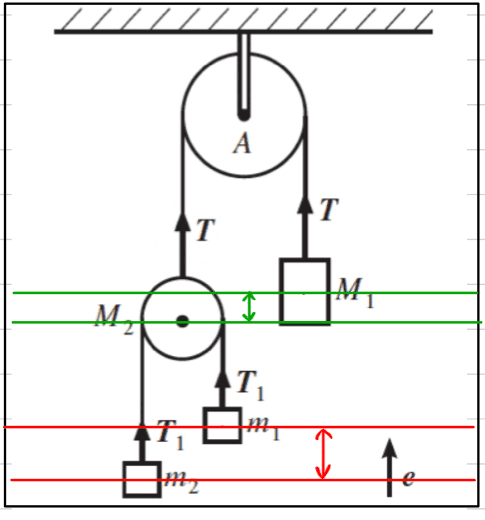Homework Helper
Gold Member
One way to test if your final formulas are correct is to put ##M_2=0## and see if they give the expected results.
If ##M_2=0## then the expected results are ##T=2T_1##, ##a_1=-a_2## and $$T_1=\frac{2gm_1m_2}{m_1+m_2}$$